# Derivation, logical

(diff) ← Older revision | Latest revision (diff) | Newer revision → (diff)
If the axioms and the rules of logical transitions are given (cf. Derivation rule), one says that the sequence of formulas is a derivation (of its last term) from the hypotheses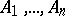(), if each term of the sequence is an axiom, is one of the hypotheses or else may be obtained from the preceding formulas in accordance with one of the rules. This is written as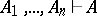. Formulais then called derivable from. If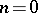, the notation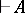means thatis derivable in the relevant calculus without any assumptions; the notation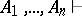, which means that "the assumptions A1…An lead to a contradiction" , is also employed (in most systems being studiedmeans that any formula at all may be deduced from these hypotheses). For instance, in a calculus containing the axiom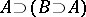and the rule of modus ponens, the sequence,,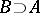, is a derivation offrom(i.e.). The following are properties of logical derivability: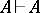; if, then; if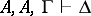, then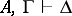; if, then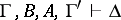; ifand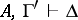, then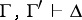(hereandare formulas,and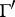are lists of formulas andis a formula or the empty word). These formulas make a substantial transformation of the list of hypotheses possible and, in conjunction with the rules of introduction and elimination of logical symbols (cf. Derived rule; Deducible rule), render systems with the symbolmuch like a Gentzen formal system. In calculi based on classical logic, a characteristic property is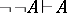. In intuitionistic logic (constructive logic) it is possible to demonstrate, under certain assumptions on, the principles of Brouwer's concept of derivability: 1) if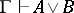, then either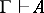or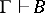; and 2) if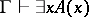, then, for some term,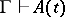(these assumptions are invariably met for the empty). Possibilities of discarding certain assumptions, including transitions to conclusions without any hypotheses, are governed by a deduction theorem.